# Polynomial Construction from Sequences VI

It's time to revisit the concept of taking differences of numbers. But we aren't dealing with numbers anymore. We are dealing with points.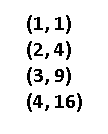That's our familiar f(x) = x^2 function. We'll use that as our first goal, but for now I'm going to explore a more familiar path when it comes to finding polynomials of points.

Remember the slope equation you may have learned in school? It's sometimes called "rise over run", or (y2 - y1)/(x2 - x1). It's how we find the slope of a linear equation using any two points. When we were performing operations over a sequence of numbers, those differences we kept taking is really the same idea: what is the change, the slope between two points. The difference here is that we must take the change in x into account: before we assumed the change in x was always 1 because x could only go up by 1 per term in a sequence. Below is the following process for finding a solution to the two-point problem.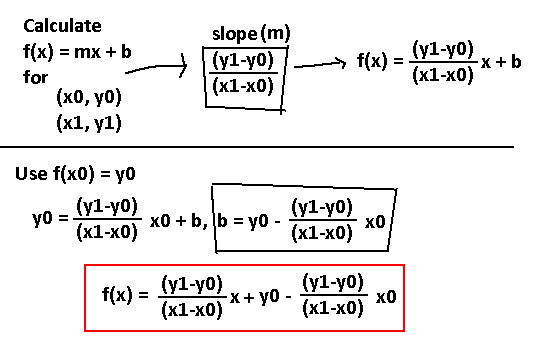You plug in any two points, and you'll get back an equation whose line passes through them, as long as they're good values (don't do something dumb like (0, 3) and (0, 4): two outputs per input isn't allowed). Notice that if you set x0 = 1 and x1 = 2 then it will reduce back to -y0(x-2) + y1(x-1), or -y0x + y1x + 2y0 - y1, our two term sequence formula!

Preserving this concept of taking these slopes between points, can we apply it to our multi-point problem? The struggle of applying this eventually led to a working concept of how we tackle more than two points.

The way we perform a difference is going to be a little… different. Instead of taking the difference between two consecutive points, we are going to take a difference between every other point and the very first point. Below is a demonstration.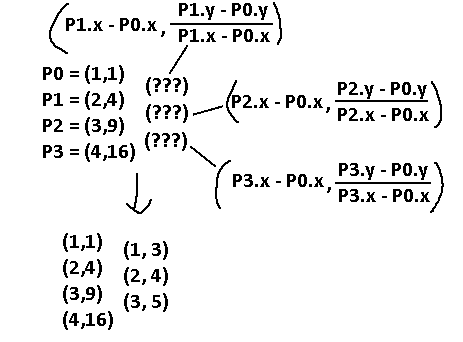We want the slope between points 0 and 1, 0 and 2, 0 and 3, instead of between 0 and 1, 1 and 2, 2 and 3. These slope values are to be used as the new y values where the (???) marks are on the image. As for the x values, they are simply the difference between the x values of the points. If we did the difference between consecutive points, we would have ended up with all of the x values being the same, 1. That makes future computations impossible, as the points we have generated all have the same x value, and we cannot have multiple outputs per one input. With this new method, what we get out of this is (1, 3), (2, 4), (3, 5), which looks like another set of well-behaved, standalone points. In fact, we are going to use them to perform this difference method a second time.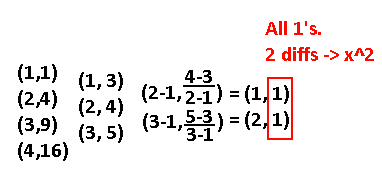We end up with some points whose y values are constant! This is very similar in our old method where we stopped after finding no more differences between numbers. Our final value is one, and since we took two differences to get to this point, this must be related to the x^2 term. In fact, this IS the x^2 term's coefficient. Turns out when using this method, the need to divide this value by some factorial number is gone.

We only used this method for a nice set of points. What happens if I, for example, mix everything up?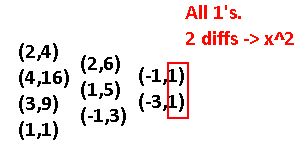Oh… wow, okay. How about a real example? One with some extra steps involved? Let's reverse engineer 2x^3 - 3x^2 + 4 using only the points (0,4), (2,8), (3,31), (5,179), and (8,836). Begin!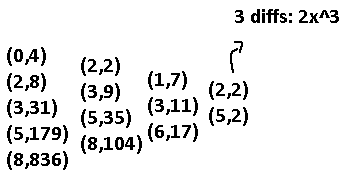I'm not going to put in the intermediate steps of calculating the slope and all that anymore. So, we got 2x^3 out of that. The y-value that is constant becomes the coefficient and we took three differences, so x^3. What now? Well, just like in the old method, we gotta subtract the original sequence by this 2x^3 for certain points. We want to subtract just the y values by 2x^3, and x is going to be the x value of that specific point. Makes sense, right?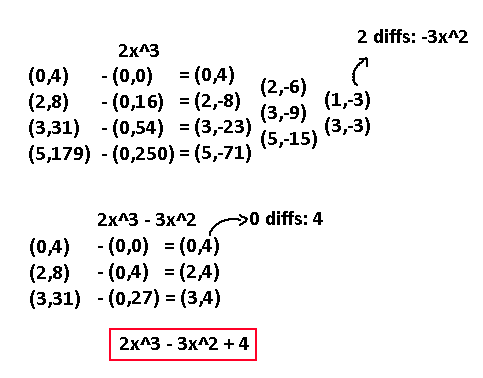Looks like it's working!….. You know exactly what's going to happen next. Find a formula for any two points.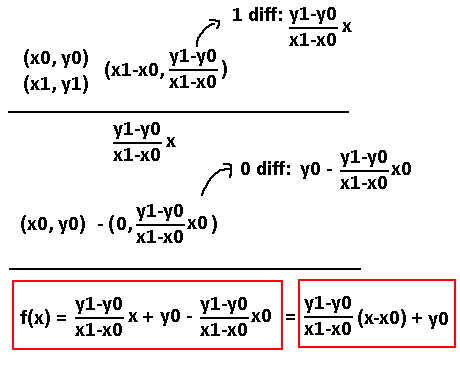Hey, that formula's back! This is understandably more complex than the old two-term formula. This makes me worry for the three-term one now…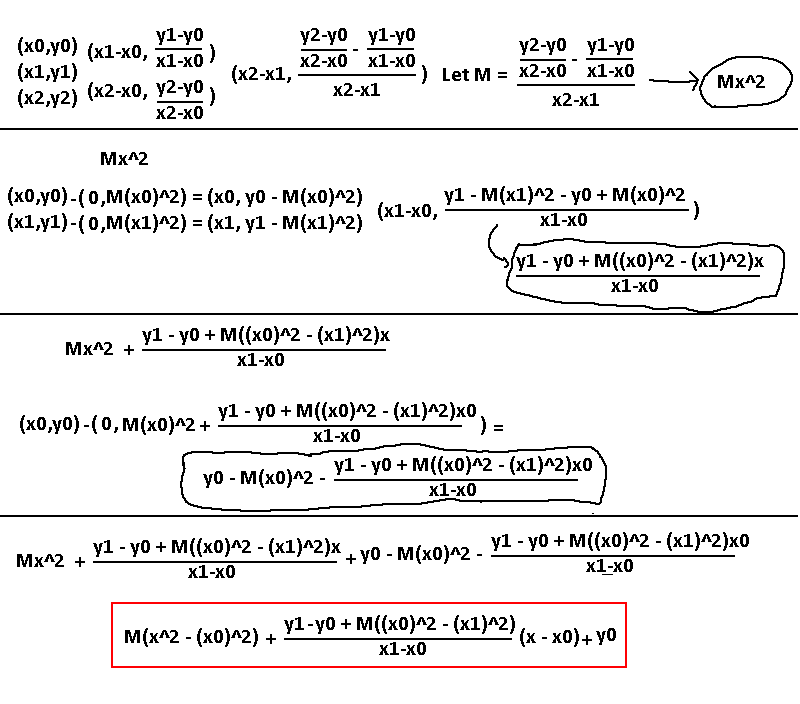Yikes. And that's with shrinking it a bit by setting that one large fraction to M. Anyways, I tried plugging in (2,0), (4,14), (5,24) and I get back x^2 + x - 6 which is correct. What a mess of algebra. It reminds me of when we grouped up terms by x before, which led us into similar monstrosities…

Before we dig into this rabbit hole, let's go back and review the first result we got, the one for the two points. Just like how we reorganized the terms in the old method to be grouped up by the constants CN, let's reorganize the terms here to be grouped up by yN values and see what happens.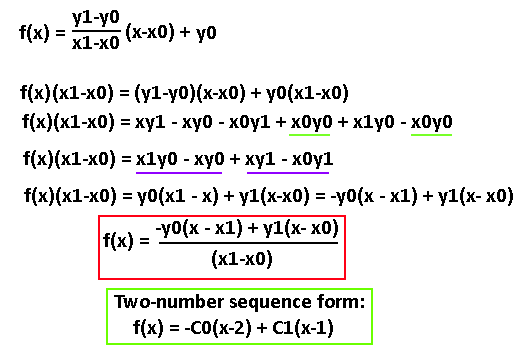The lines mean a couple of terms could cancel each other out or combine. Does the result in the red box look familiar? I put the old 2-term equation right underneath for a comparison. Now we see how they're truly related! When setting x0 = 1 and x1 = 2, that's why our old 2-term equation had (x-1) and (x-2) in there! And the denominator in the red box would have been (2-1) = 1, which is why the green box has no denominator.

If we haven't gone through the tedious work of finding the simpler forms for an N-number sequence, it would've been much more difficult to tackle this generalized problem. But, now we have a basic understanding of what the three-point equation should look like, and we can use our old formulas as a reference to guide us to a correct result. Let's now go back to figuring out the three-point equation, the smart way.

Here's our three-number sequence, for comparison. I left the letters as letters; it won't matter to us.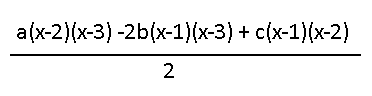Let's try using this expression as a template to help us build the generic three-point solution. We know that each of the letters is really just a certain y value. It should be a safe bet to replace a with y0, b with y1, and c with y2. Additionally, in the parentheses those constants will likely be replaced with our generic xN terms. In the two-point form what basically happened was 1 was replaced by x0, and 2 was replaced by x1. Let's do that here, only replace 3 by x2.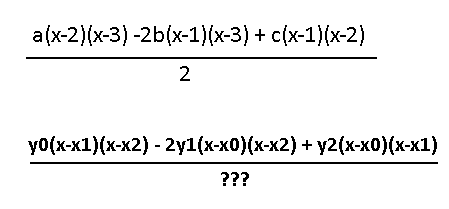Almost ready to test it! Now what goes in the denominator? In the two-term form there was a (x1-x0) factor. Maybe in this one there are multiple factors which include x0, x1, and x2 now? Whatever the expression is, we should be able to input x0 = 1, x1 = 2, and x2 = 3 and get out a 2, since 2 is what comes out of the two-number sequence form. Maybe it's just (x2-x0)? That would get us two. The factors (x2-x1) and (x1-x0) could also be in there, since they both evaluate to 1. How about we just leave this as is, and try plugging in three points we know the equation to, and see what we get? Not every solution needs a rigid explanation behind it; for now we just care about the emerging patterns. Let's bring back (2,0), (4,14), (5,24), which was x^2 + x - 6. Plugging the values in we get… -4x^2 + 52x - 88. Wow that's not even close to right. Of course it wasn't that easy.

Looks like there's not much choice but to try and simplify the 3-point form like we did the two-point form. I am not digitizing my work to show the results here. It almost took an entire page of algebra to extract out anything reducible. I'll show a photo of my paper instead, so you can witness what I had to go through: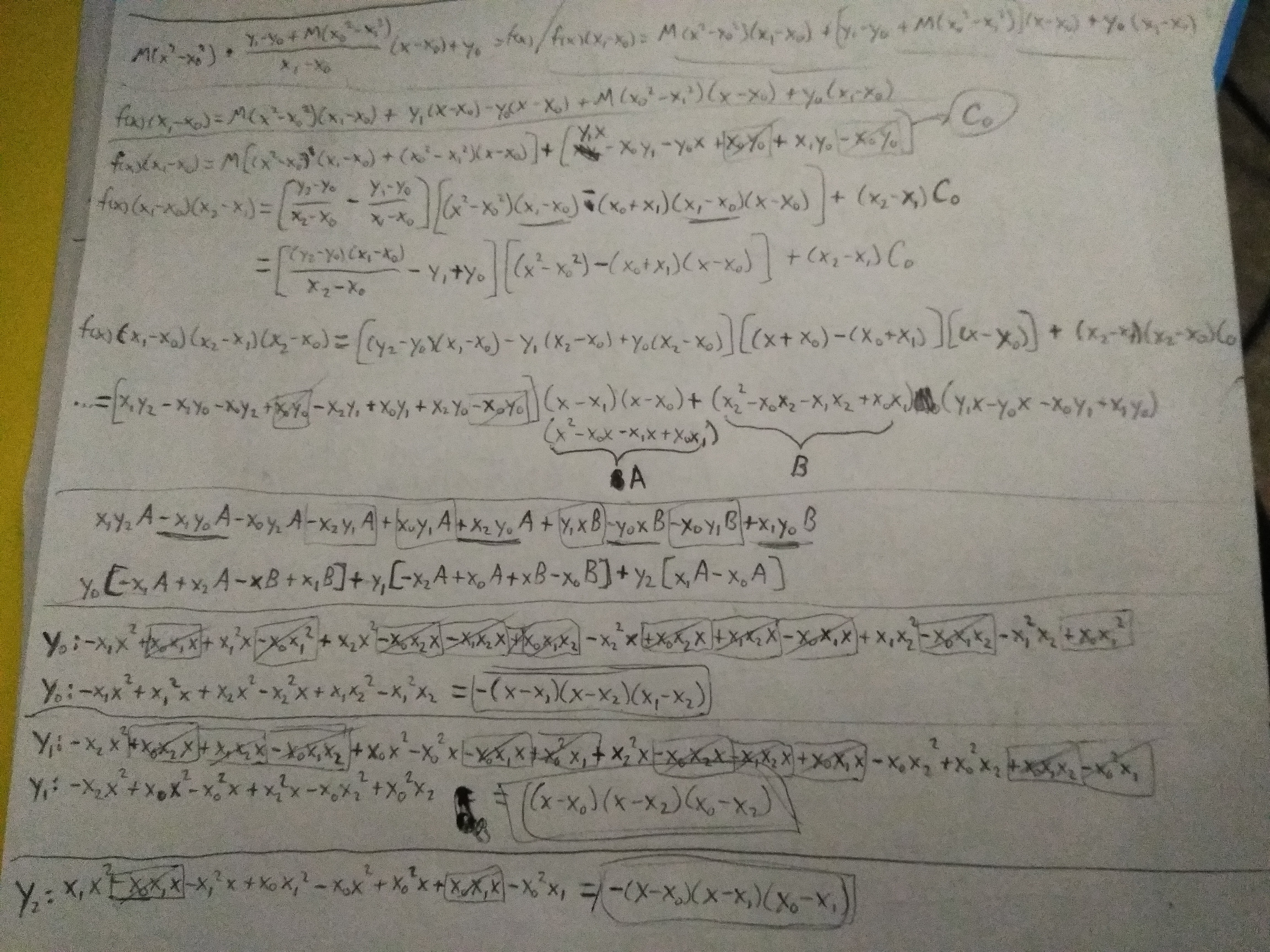I figured noone would mind the brightness, and just vaguely skim through the process of factoring out all the y-terms. Anyway, what we're left with is this: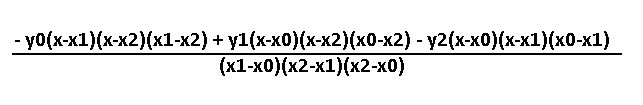Notice how it's different from our original guess. Each y value has an extra term multiplied to it, and the coefficients are slightly different; the coefficients are also likely generalized, and those extra terms are meant to generate that coefficient since those terms are constants. Plug in x0 = 1, x1 = 2, and x2 = 3 and see for yourself! I'm going to try plugging in (2,0), (4,14), (5,24) again and see what happens.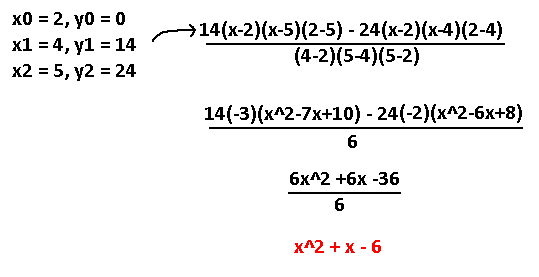n i c e

I think this is a nice victory to stop on. I'm going to finish this entire thing off on the next post. We're getting very close to a true solution to the N-point polynomial problem.